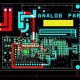# Basic Digital Electronics

In this tutorial we are going to learn about Basic Digital Electronics:

• Number System & Codes
• Logic Gates
•  Boolean Algebra
• Combinational Circuit
• Sequential Circuit
• Logic Families
• Semiconductor Memories
• A/D & D/A Converters
• Micro Processor

Number System & Codes – Number systems and binary codes. The binary system is a number system, where the numbers are represented as the digits 0 and 1and which is used in the computers, while they work with two voltage levels, and also their natural number system is the binary system means of (1 – ON, 0 – OFF)

Logic gates – These are the basic building blocks of digital system. It is electronic circuit which having one or more than one input and only one output and there is relationship between the input and the output is based on certain logic. These are Based on this, logic gates are named as AND gate, OR gate, NOT, NOR gate, X-OR, X-NOR & NAND gate.

Boolean algebra – In mathematical logic, It is the branch of algebra in which the values of the variables are the truth values true and false, generally denoted 1 and 0.

Combinational Circuits – This is the circuit which is made up of different types of logic gates and logic gate is a basic building block of any electronic circuit. Usually the output of the combinational circuit depends on the values at the input at any given time. The circuits do not make use of any memory or storage device.

Sequential Circuit – A Sequential circuit combinational logic circuit which consists of inputs variable (X), logic gates (Computational circuit), and output variable (Z). Here Combinational circuit an output based on only input variable, but Sequential circuit produces an output based on current input and previous input variables.

Logic family – A logic families of monolithic digital integrated circuit devices is a group of electronic logic gates that is constructed using one of several different designs, usually with compatible logic levels and power supply characteristics within a family.

Semiconductor Memories – It is a digital electronic semiconductor device which is used for digital data storage, such as computer memory and it contrasts with data storage media like that hard disks and CD which read and write data consecutively and therefore the data can only be accessed in the same sequence it was written.

A/D & D/Converters – A/D means analog to digital converter and digital to analog converter. Analog signals are directly measurable quantities. Digital signals only have two states. For digital computer, It is refer to binary.

Micro Processor – It is an electronic component which is used by a computer to do its work and it is a central processing unit on a single integrated circuit chip which containing millions of very small components including transistors, resistors, and diodes that work together.## About EEE

We have designing Experience for the last 40 years.